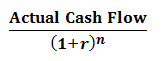# Discounted Payback Period

Discounted Payback Period is the time required to recover the original cost of an investment from the net cash flows considering the time value of money. It is an enhanced version of the Payback Period where the time value of money is not considered.

• X = Last period with a negative discounted cumulative cash flow
• Y =Value of discounted cumulative cash flow at the end of the period X
• Z = Discounted cash flow during the period after X.
Discounted Cash Flow per Period=(r is the rate of interest and n is number of years)
• An Investment with Shorter Discounted Payback Period is preferred over an investment with Longer Payback Period.
A shorter discounted payback period is more favorable. While comparing two investments having similar returns, the one with a shorter payback period is to be considered.
Illustration 1: XYZ Ltd has invested Rs. 25000 in a project that promises net cash flows in the following order, calculate the Discounted Payback Period for ABC Ltd. if the rate of interest is 10%.
 Year Year 1 Year 2 Year 3 Year 4 Year 5 Net Cash Flow 5000.00 8000.00 9000.00 8000.00 12000.00
Solution:
In such cases where the cash flow is uneven, the following steps are followed to calculate the Discounted Payback period
• Step 1: Create a table of the net cash flow and the cumulative flow. Cumulative flow is the net cash balance after adjusting the net cash flow. The initial investment amount can be considered as a negative cash flow. The initial point i.e. year 0, will have a negative balance equal to the amount invested initially.
 Year Net Cash Flow Discounted Cash Flow Cumulative Flow 0 -25000 0 -25000 1 5000 4545.45 -20454.45 2 8000 6611.57 -13842.88 3 9000 6761.83 -7081.05 4 8000 5464.11 -1616.94 5 12000 7451.06 5834.12
• Step 2: Select the year which has the last negative cumulative flow. In above case it will be 4thYear.
• Step 3: Divide the Cumulative Flow of the year obtained in Step 2 by the discounted cash flow of the immediate next year. (Ignore the sign)
1616.94/7451.06 = 0.217
• Step 4: The answer is the sum of Step 2 and Step 3 i.e. 4.217 Years.
Hence, Discounted Payback Period of above cashflow is 4.217 years